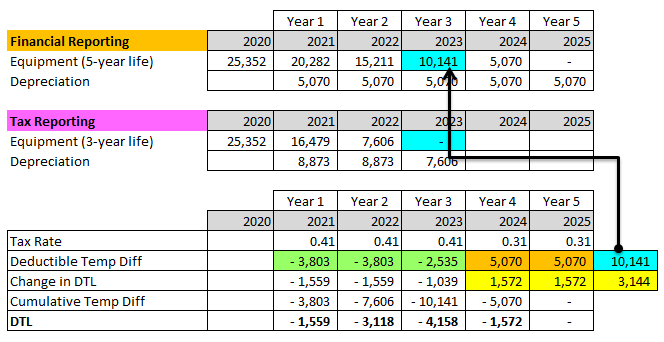# Calculate deferred tax liability

Hi everyone,

I’m going through some quizzes I found online for the Income Taxes Chapter. I taught I mastered the chapter, but I got a mediocre result on the end of chapter questions, so I turned to some additional quizzes found online. I know this kind of resource can be of doubtful reliability and accuracy, so I would need your help.

One quiz goes like: a company buys new equipment with 5 yrs useful life for 25,352. For accounting purposes the asset is depreciated with the straight line method, while for tax purposes it’s depreciated of 35% of its historic cost for the first 2 years and 30% of its historic cost on year 3. Annual revenues of the company are 14,384 constant over the next 5 years. The tax rate will change on years 4 and 5 from 41% to 31%. What is the deferred tax liability at the end of year 3?

I personally would have solved this by calculating the carrying amount and tax base, taking the difference and multiplying it by 41% to get a DTL of 4,158.

However, on the solutions the depreciation for accounting and tax purposes is calculated, then for each of the 3 years the difference between the income and the depreciation is calculated (again for tax and reporting purposes), then the cumulative difference between the accounting and tax reporting incomes for 3 years is calculated and, finally this difference is multiplied for the 31% tax rate to get to a DTL of 3,144. The whole process shown in the solution doesn’t make any sense to me. Also, I believe the tax rate of 41% should be used, given that the change in tax rate will occur in Y4 and Y5.

Am I missing something? Or is the solution actually correct?

Thanks in advance to anyone who will try and help me on this.

The only difference between your approach and theirs is the tax rate; if you had used a 31% tax rate you would have gotten 3,144 as they did.

The correct rate to use is 31%, because that’s the rate that will apply when the remainder of the difference in carrying amounts is resolved: in years 4 and 5.

The answer is correct.
The way to solve this is to simply get the carrying value at end of Year 3 as it is already fully tax depreciated. Apply the expected tax rate: 10,141 x 31% = 3,144.(see yellow cells in the image below).

Tax Rate from Standards:
IFRS (use enacted or substantively enacted by the end of the reporting period)
US GAAP (use enacted tax rate expected to apply)

Here is the lifetime accounting of DTL: